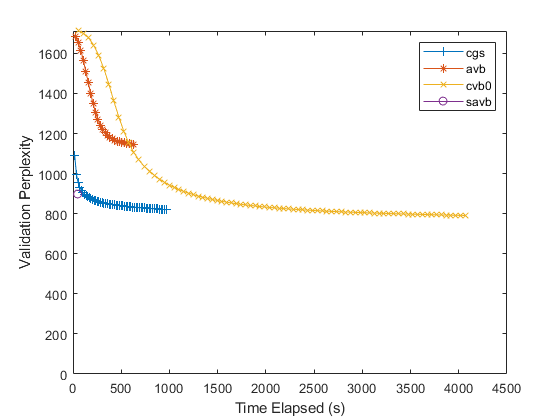# Compare LDA Solvers

This example shows how to compare latent Dirichlet allocation (LDA) solvers by comparing the goodness of fit and the time taken to fit the model.

### Import Text Data

Import a set of abstracts and category labels from math papers using the arXiv API. Specify the number of records to import using the `importSize` variable.

`importSize = 50000;`

Create a URL that queries records with set `"math"` and metadata prefix `"arXiv"`.

```url = "https://export.arxiv.org/oai2?verb=ListRecords" + ... "&set=math" + ... "&metadataPrefix=arXiv";```

Extract the abstract text and the resumption token returned by the query URL using the `parseArXivRecords` function which is attached to this example as a supporting file. To access this file, open this example as a live script. Note that the arXiv API is rate limited and requires waiting between multiple requests.

`[textData,~,resumptionToken] = parseArXivRecords(url);`

Iteratively import more chunks of records until the required amount is reached, or there are no more records. To continue importing records from where you left off, use the resumption token from the previous result in the query URL. To adhere to the rate limits imposed by the arXiv API, add a delay of 20 seconds before each query using the `pause` function.

```while numel(textData) < importSize if resumptionToken == "" break end url = "https://export.arxiv.org/oai2?verb=ListRecords" + ... "&resumptionToken=" + resumptionToken; pause(20) [textDataNew,labelsNew,resumptionToken] = parseArXivRecords(url); textData = [textData; textDataNew]; end```

### Preprocess Text Data

Set aside 10% of the documents at random for validation.

```numDocuments = numel(textData); cvp = cvpartition(numDocuments,'HoldOut',0.1); textDataTrain = textData(training(cvp)); textDataValidation = textData(test(cvp));```

Tokenize and preprocess the text data using the function `preprocessText` which is listed at the end of this example.

```documentsTrain = preprocessText(textDataTrain); documentsValidation = preprocessText(textDataValidation);```

Create a bag-of-words model from the training documents. Remove the words that do not appear more than two times in total. Remove any documents containing no words.

```bag = bagOfWords(documentsTrain); bag = removeInfrequentWords(bag,2); bag = removeEmptyDocuments(bag);```

For the validation data, create a bag-of-words model from the validation documents. You do not need to remove any words from the validaiton data because any words that do not appear in the fitted LDA models are automatically ignored.

`validationData = bagOfWords(documentsValidation);`

### Fit and Compare Models

For each of the LDA solvers, fit a model with 40 topics. To distinguish the solvers when plotting the results on the same axes, specify different line properties for each solver.

```numTopics = 40; solvers = ["cgs" "avb" "cvb0" "savb"]; lineSpecs = ["+-" "*-" "x-" "o-"];```

Fit an LDA model using each solver. For each solver, specify the initial topic concentration 1, to validate the model once per data pass, and to not fit the topic concentration parameter. Using the data in the `FitInfo` property of the fitted LDA models, plot the validation perplexity and the time elapsed.

The stochastic solver, by default, uses a mini-batch size of 1000 and validates the model every 10 iterations. For this solver, to validate the model once per data pass, set the validation frequency to `ceil(numObservations/1000)`, where `numObservations` is the number of documents in the training data. For the other solvers, set the validation frequency to 1.

For the iterations that the stochastic solver does not evaluate the validation perplexity, the stochastic solver reports `NaN` in the `FitInfo` property. To plot the validation perplexity, remove the NaNs from the reported values.

```numObservations = bag.NumDocuments; figure for i = 1:numel(solvers) solver = solvers(i); lineSpec = lineSpecs(i); if solver == "savb" numIterationsPerDataPass = ceil(numObservations/1000); else numIterationsPerDataPass = 1; end mdl = fitlda(bag,numTopics, ... 'Solver',solver, ... 'InitialTopicConcentration',1, ... 'FitTopicConcentration',false, ... 'ValidationData',validationData, ... 'ValidationFrequency',numIterationsPerDataPass, ... 'Verbose',0); history = mdl.FitInfo.History; timeElapsed = history.TimeSinceStart; validationPerplexity = history.ValidationPerplexity; % Remove NaNs. idx = isnan(validationPerplexity); timeElapsed(idx) = []; validationPerplexity(idx) = []; plot(timeElapsed,validationPerplexity,lineSpec) hold on end hold off xlabel("Time Elapsed (s)") ylabel("Validation Perplexity") ylim([0 inf]) legend(solvers)```For the stochastic solver, there is only one data point. This is because this solver passes through input data once. To specify more data passes, use the `'DataPassLimit'` option. For the batch solvers (`"cgs"`, `"avb"`, and `"cvb0"`), to specify the number of iterations used to fit the models, use the `'IterationLimit'` option.

A lower validation perplexity suggests a better fit. Usually, the solvers `"savb"` and `"cgs"` converge quickly to a good fit. The solver `"cvb0"` might converge to a better fit, but it can take much longer to converge.

For the `FitInfo` property, the `fitlda` function estimates the validation perplexity from the document probabilities at the maximum likelihood estimates of the per-document topic probabilities. This is usually quicker to compute, but can be less accurate than other methods. Alternatively, calculate the validation perplexity using the `logp` function. This function calculates more accurate values but can take longer to run. For an example showing how to compute the perplexity using `logp`, see Calculate Document Log-Probabilities from Word Count Matrix.

### Preprocessing Function

The function `preprocessText` performs the following steps:

1. Tokenize the text using `tokenizedDocument`.

2. Lemmatize the words using `normalizeWords`.

3. Erase punctuation using `erasePunctuation`.

4. Remove a list of stop words (such as "and", "of", and "the") using `removeStopWords`.

5. Remove words with 2 or fewer characters using `removeShortWords`.

6. Remove words with 15 or more characters using `removeLongWords`.

```function documents = preprocessText(textData) % Tokenize the text. documents = tokenizedDocument(textData); % Lemmatize the words. documents = addPartOfSpeechDetails(documents); documents = normalizeWords(documents,'Style','lemma'); % Erase punctuation. documents = erasePunctuation(documents); % Remove a list of stop words. documents = removeStopWords(documents); % Remove words with 2 or fewer characters, and words with 15 or greater % characters. documents = removeShortWords(documents,2); documents = removeLongWords(documents,15); end```